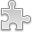# Courses / Module

Toggle Print

##INTRODUCTION TO STATISTICS (S)

Module code: ST221
Credits: 5
Semester: 1
Department: MATHEMATICS AND STATISTICS
International:Overview

Probability: Sample spaces, events, counting methods, conditional probability, independence, Bayes theorem. Discrete random variables, mean and variance. Binomial and Poisson. Continuous random variables. The Normal model. Bivariate discrete random variables. Covariance and correlation. Sampling distribution of the mean. Confidence intervals and hypothesis testing: one and two samples, paired samples. Confidence intervals and hypothesis testing for one proportion. Computation using statistical software.Learning OutcomesTeaching & Learning methodsAssessmentAutumn Supplementals/ResitsPre-RequisitesTimetable